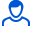Content: teorver_5585.doc (28.00 KB)

Positive responses: 0
Negative responses: 0

Refunds: 05585. x̅=114.36, n=81, σ=9. Find the confidence interval for estimating with a reliability of 0.99 the unknown mathematical expectation a of the normal distributed feature X of the general population, knowing the sample mean x̅=114.36, the sample size n=81 and the standard deviation σ=9.
Detailed solution. Decorated in Microsoft Word 2003 (Quest decided to use the formula editor)
No feedback yet